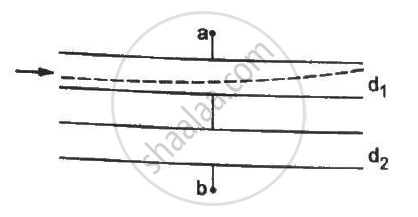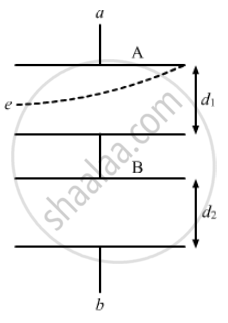Advertisement Remove all ads

# Both the Capacitors Shown in Figure Are Made of Square Plates of Edge A. the Separations Between the Plates of the Capacitors Are D1 and D2 as Shown in the Figure. a Potential Difference - Physics

Sum

Both the capacitors shown in figure are made of square plates of edge a. The separations between the plates of the capacitors are d1 and d2 as shown in the figure. A potential difference V is applied between the points a and b. An electron is projected between the plates of the upper capacitor along the central line. With what minimum speed should the electron be projected so that it does not collide with any plate? Consider only the electric forces.Advertisement Remove all ads

#### SolutionLet

Velocity of the electron = u

Mass of the electron = m

Now,

Horizontal distance, x = u × t

⇒ t = x/v  ..........(1)

Let the electric field inside the capacitor be E

∴ Acceleration of the electron = (qE)/m

Vertical Distance,  y = 1/2 (qE)/mt^2 = 1/2 (qE)/m(x/u)^2  ..........(∵ t = x/u)

y = d_1/2 and x = a

⇒ d_1/2 = 1/2 (qE)/m . (a/u)^2       .............(2)

Capacitance of the two capacitors :-

C_1 = (∈_0a^2)/(d_1) and C_2 = (∈_0a^2)/(d_2)

It is given that the capacitors are connected in series.

Thus, the equivalent capacitance is given by

C_(eq) = (C_1C_2)/(C_1+C_2)

C_(eq) = ((∈_0a^2)/d_1 xx (∈_0a^2)/d_2)/((∈_0a^2)/(d_1)+(∈_0a^2)/(d_2)) = (∈_0a^2)/((d_1+d_2))

Total charge on the system of capacitors, Q = C_(eq)V =(∈_0a^2)/((d_1+d_2))V

As the capacitors are in series, charge on both of them is the same.

The potential difference across the capacitor containing the electron is given by

V = Q/C_1 = (∈_0a^2V)/(C_1(d_1+d_2) = (∈_0a^2V)/(((∈_0a^2)/d_1)(d_1+d_2)) = (Vd_1)/(d_1+d_2)

The magnitude of the electric field inside the capacitor is given by E = V/d_1 = V/(d_1+d_2)

The charge on electron q is represented by e.

On putting the values of q and E in (2), we get

⇒ d_1/2 = 1/2 (qV)/(m(d_1+d_2)) . (a/u)^2  ..........(3)

The minimum velocity of the electron is given by

u = ((Vea^2)/(md_1(d_1+d_2)))^(1/2)

Is there an error in this question or solution?
Advertisement Remove all ads

#### APPEARS IN

HC Verma Class 11, Class 12 Concepts of Physics Vol. 2
Chapter 9 Capacitors
Q 22 | Page 166
Advertisement Remove all ads

#### Video TutorialsVIEW ALL 

Advertisement Remove all ads
Share
Notifications

View all notifications

Forgot password?